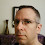## Monday, July 4, 2011

### Difference between \$x and \${x}

Many times in shell scripts we would have witnessed two different forms of notations being used against variables: \$x and \${x}.  Are they both same or is there any difference between them? Let us discuss in this article:

Let us assign a value to the variable, x:
```\$ x=10
```

Now, lets try to display the value using both the above notations:
```\$ echo \$x
10
```
Displaying using the second notation:
```\$ echo \${x}
10
```
As seen above, it does not look like there is any difference. But it is not so. The actual difference we will see now:

Let us try to join or concatenate the literal "y" along with the value of \$x:
```\$ echo \$xy

```
Nothing got printed for "echo \$xy".  Now, let us the second notation to display:
```\$ echo \${x}y
10y
```
What happened? When we said \$xy, the shell interpreted it as being asked to print the value of a variable named "xy". Since there is no such variable "xy" being defined, a blank output. However, the second notation \${x}y is being interpreted as : print the value of x and concatenate it with the literal "y", and hence the result "10y".

1.2.